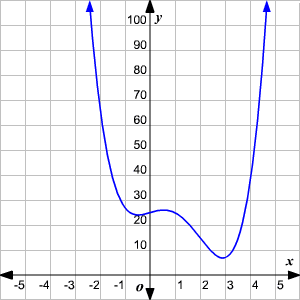# End Behavior of a Function

The end behavior of a polynomial function is the behavior of the graph of $f\left(x\right)$ as $x$ approaches positive infinity or negative infinity.

The degree and the leading coefficient of a polynomial function determine the end behavior of the graph.

The leading coefficient is significant compared to the other coefficients in the function for the very large or very small numbers. So, the sign of the leading coefficient is sufficient to predict the end behavior of the function.

 Degree Leading Coefficient End behavior of the function Graph of the function Even Positive Example: $f\left(x\right)={x}^{2}$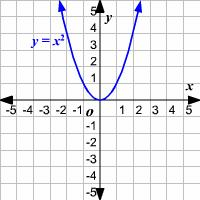Even Negative Example: $f\left(x\right)=-{x}^{2}$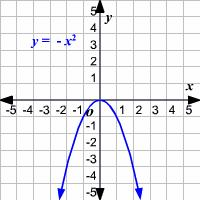Odd Positive Example: $f\left(x\right)={x}^{3}$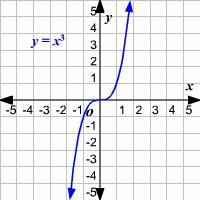Odd Negative Example: $f\left(x\right)=-{x}^{3}$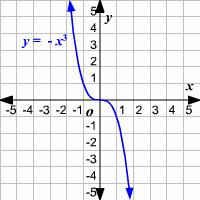To predict the end-behavior of a polynomial function, first check whether the function is odd-degree or even-degree function and whether the leading coefficient is positive or negative.

Example:

Find the end behavior of the function ${x}^{4}-4{x}^{3}+3x+25$.

The degree of the function is even and the leading coefficient is positive. So, the end behavior is:

The graph looks as follows: